Using 2 ultrasonic sensors to detect the strum motion. And using 3 buttons to play the node

IntermediateShowcase (no instructions)258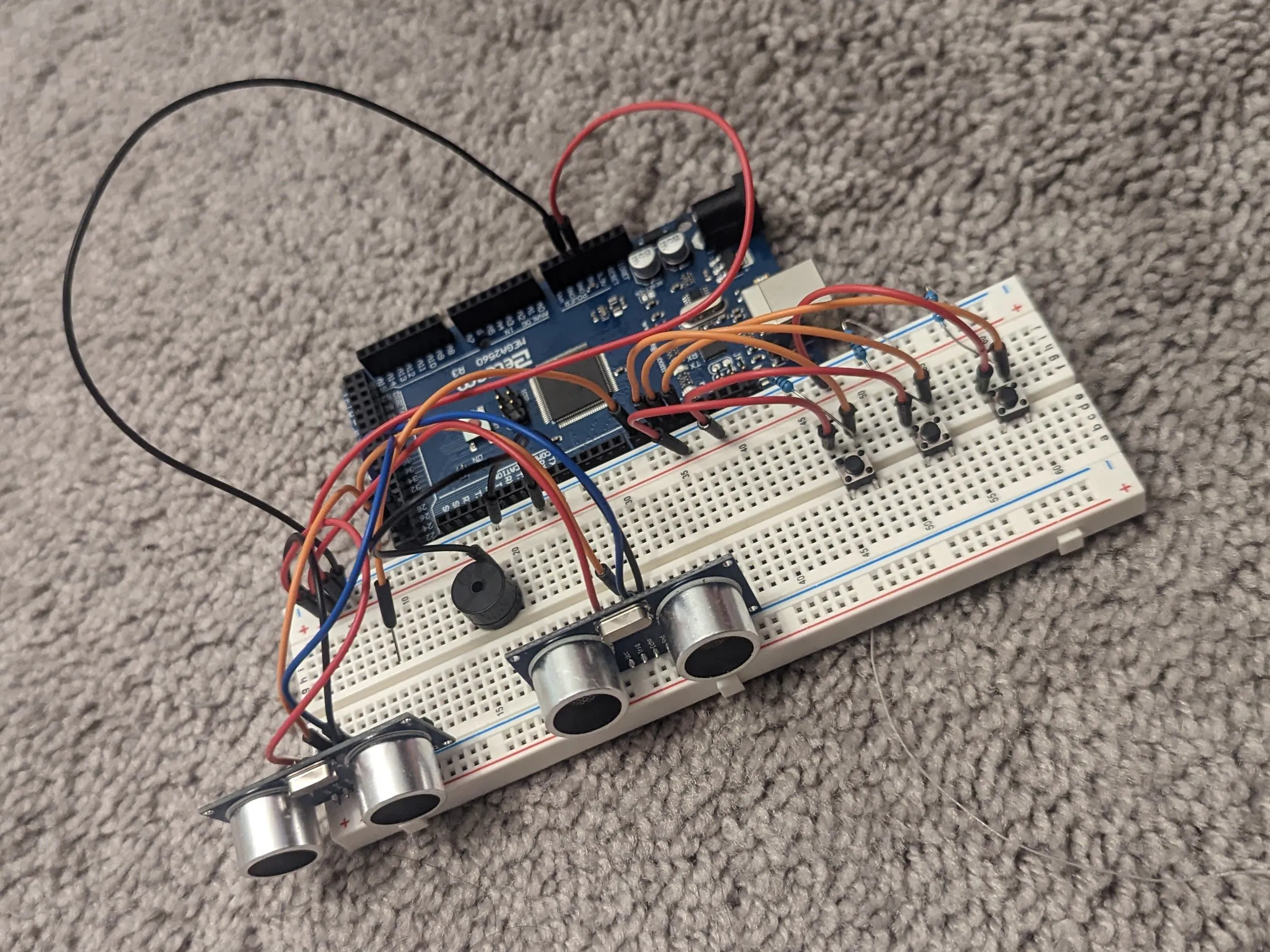## Things used in this project

### Hardware components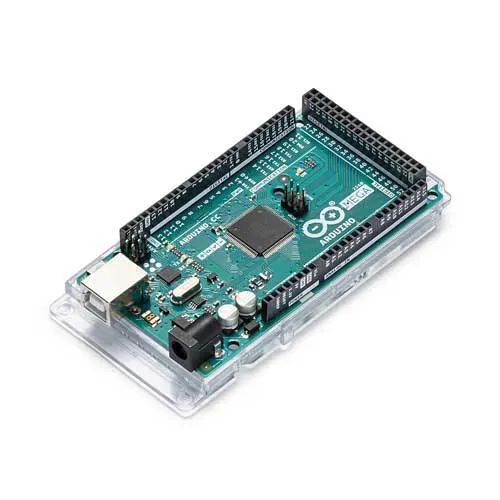Arduino Mega 2560
×1Ultrasonic Sensor - HC-SR04 (Generic)
×2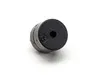Buzzer
×1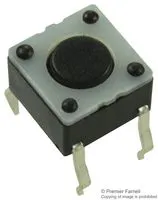Tactile Switch, Top Actuated
×1

### Software apps and online servicesArduino IDE

## Schematics

### CIRCUIT

I am using Mega2560. But in the image is Arduino UNO. The port number is not correct. The code is the correct.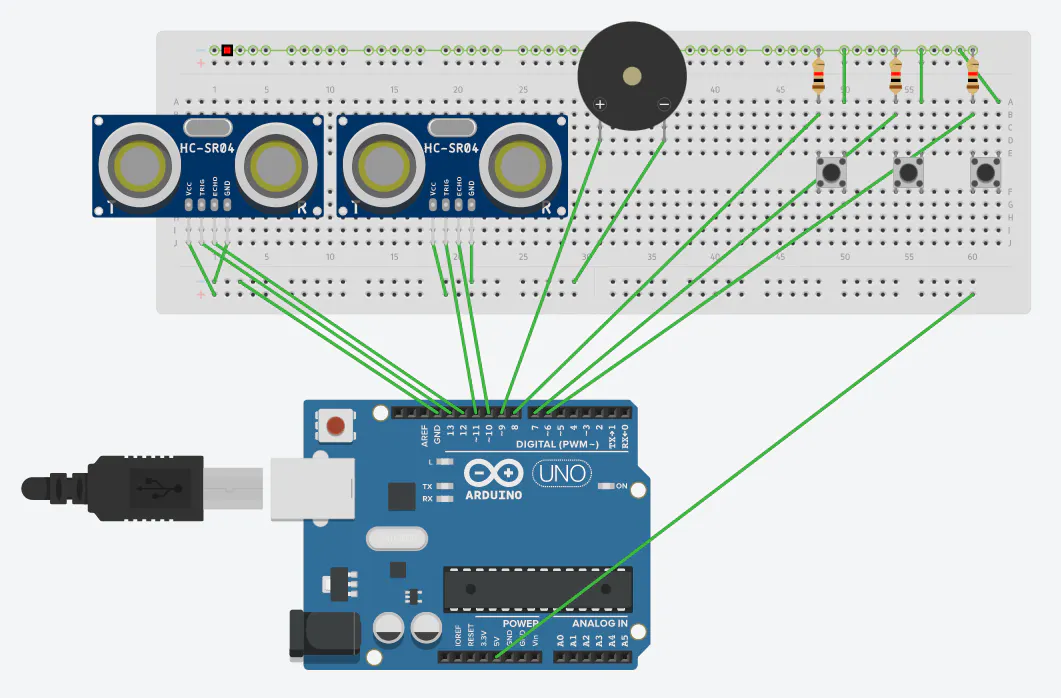## Code

### Untitled file

Arduino
```#include "pitches.h"  //add note library

#define trigPin2 23 // pin for sending sensor #1 trigger signal
#define echoPin2 22 // pin for receiving sensor #1 echo signal
#define trigPin1 25  // pin for sending sensor #2 trigger signal
#define echoPin1 24  // pin for receiving sensor #2 echo signal

//digital pin 12 has a button attached to it. Give it an name
int buttonPin1= 12;
int buttonPin2= 11;
int buttonPin3= 10;

int buzzerPin = 8;

// defines variables
long duration1; // variable for the duration of sound wave travel
long duration2;
int distance1; // variable for centimeters measurement
int distance2; // variable for inches measurement

long d1;                  // sensor 1 distance
long d2;                  // sensor 2 distance
long t1 = 0;              // sensor 1 timestamp; initialize as 0
long t2 = 0;              // sensor 2 timestamp; initialize as 0
unsigned long start_time; // time since program start
float max_distance = 15;  // movement sensing range (in cm)
float min_distance =3;

void setup() {
//make the button's pin input
pinMode(buttonPin1, INPUT);
pinMode(buttonPin2, INPUT);
pinMode(buttonPin3, INPUT);

pinMode(trigPin1, OUTPUT); // Sets the trigPin as an OUTPUT
pinMode(echoPin1, INPUT); // Sets the echoPin as an INPUT
pinMode(trigPin2, OUTPUT); // Sets the trigPin as an OUTPUT
pinMode(echoPin2, INPUT); // Sets the echoPin as an INPUT
//Serial.begin(9600); // // Serial Communication is starting with 9600 of baudrate speed

start_time = millis();  // get program start time
}
void loop() {

if (buttonState1 == 0 && buttonState2 == 0 && buttonState3 == 0) { // if left sensor triggered first
noTone(buzzerPin);     // Stop sound...
}

// Clears the trigPin condition
digitalWrite(trigPin1, LOW);
delayMicroseconds(2);
// Sets the trigPin HIGH (ACTIVE) for 10 microseconds
digitalWrite(trigPin1, HIGH);
delayMicroseconds(10);
digitalWrite(trigPin1, LOW);
// Reads the echoPin, returns the sound wave travel time in microseconds
duration1 = pulseIn(echoPin1, HIGH);

// Clears the trigPin condition
digitalWrite(trigPin2, LOW);
delayMicroseconds(2);
// Sets the trigPin HIGH (ACTIVE) for 10 microseconds
digitalWrite(trigPin2, HIGH);
delayMicroseconds(10);
digitalWrite(trigPin2, LOW);
// Reads the echoPin, returns the sound wave travel time in microseconds
duration2 = pulseIn(echoPin2, HIGH);

// Calculating the distance
distance1 = duration1 * 0.034 / 2; // Speed of sound wave divided by 2 (go and back)
distance2 = duration2 * 0.034 / 2; // Speed of sound wave divided by 2 (go and back)

d1 = distance1;
d2 = distance2;

// if either sensor distance drops below limit, record timestamp
if (d1 < max_distance && d1 > 3) {
t1 = millis();
}
else if (d2 < max_distance && d2 > 3) {
t2 = millis();
}

if (t1 > 0 && t2 > 0) {       // if both sensors have nonzero timestamps
if ((t1 < t2)) {
noTone(buzzerPin);
//Serial.println("Left to right");    // direction is left to right
if (buttonState1 == 1 && buttonState2 == 0 && buttonState3 == 0) {
tone(buzzerPin, NOTE_C4);
}
else if (buttonState1 == 0 && buttonState2 == 1 && buttonState3 == 0){
tone(buzzerPin, NOTE_D4);
}
else if (buttonState1 == 0 && buttonState2 == 0 && buttonState3 == 1){
tone(buzzerPin, NOTE_E4);
}
else if (buttonState1 == 1 && buttonState2 == 1 && buttonState3 == 0){
tone(buzzerPin, NOTE_F4);
}
else if (buttonState1 == 1 && buttonState2 == 0 && buttonState3 == 1){
tone(buzzerPin, NOTE_G4);
}
else if (buttonState1 == 0 && buttonState2 == 1 && buttonState3 == 1){
tone(buzzerPin, NOTE_A4);
}
else if (buttonState1 == 1 && buttonState2 == 1 && buttonState3 == 1){
tone(buzzerPin, NOTE_B4);
}

}

else if (t2 < t1) {
noTone(buzzerPin);               // if right sensor triggered first'
//Serial.println("Right to left");    // direction is right to left
if (buttonState1 == 1 && buttonState2 == 0 && buttonState3 == 0) {
tone(buzzerPin, NOTE_C4);
}
else if (buttonState1 == 0 && buttonState2 == 1 && buttonState3 == 0){
tone(buzzerPin, NOTE_D4);
}
else if (buttonState1 == 0 && buttonState2 == 0 && buttonState3 == 1){
tone(buzzerPin, NOTE_E4);
}
else if (buttonState1 == 1 && buttonState2 == 1 && buttonState3 == 0){
tone(buzzerPin, NOTE_F4);
}
else if (buttonState1 == 1 && buttonState2 == 0 && buttonState3 == 1){
tone(buzzerPin, NOTE_G4);
}
else if (buttonState1 == 0 && buttonState2 == 1 && buttonState3 == 1){
tone(buzzerPin, NOTE_A4);
}
else if (buttonState1 == 1 && buttonState2 == 1 && buttonState3 == 1){
tone(buzzerPin, NOTE_B4);
}
}
// else {
//   Serial.println("No movement");         // else print nothing (shouldn't happen)
// }

// after printing direction, reset both timestamps to 0
t1 = 0;
t2 = 0;
}

}
```

## Credits

### dmqlkig

0 projects • 0 followers